Courses

# Practice Worksheet: Number System- 2 Class 9 Notes | EduRev

## Class 9 : Practice Worksheet: Number System- 2 Class 9 Notes | EduRev

The document Practice Worksheet: Number System- 2 Class 9 Notes | EduRev is a part of the Class 9 Course Mathematics (Maths) Class 9.
All you need of Class 9 at this link: Class 9

Q.1. The value of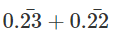is
(a) 0.45
(b)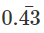(c) 45/1000
(d)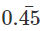Ans.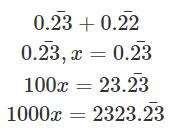9900 x = 2300
x = 23/99
Similarly x = 22/99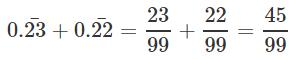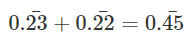Q.2. If n is a natural number, then √n is
(a)
Always a natural number
(b)
Always a rational number
(c)
Always a irrational number
(d)
Sometimes a natural number and sometimes a surd
Ans.
(d)

Q.3. If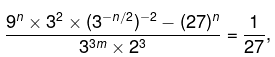prove m-n = 1
Ans.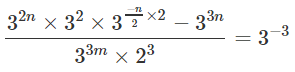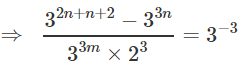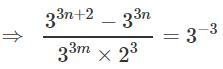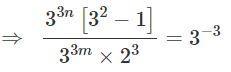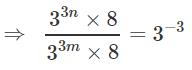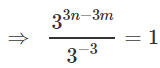⇒ 33n - 3m +3 = 30
⇒ 3n - 3m +3 = 0
⇒ n - m +1 = 0
⇒ m- n = 1

Q.4. The value of  ((23 + 22)2/3 + (140 - 29)1/2)2 is
(a)
196
(b)
286
(c)
400
(d)
324
Ans.
(c)
((23 + 22)2/3 + (140 - 29)1/2)2
(9+11)2  = 20 = 400
Hence option (c) is correct.

Q.5. If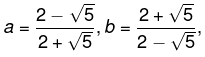find a2 - b, a+ b
Ans.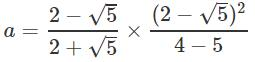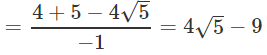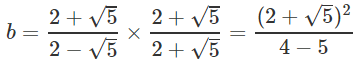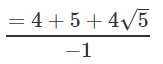= -9 - 4√5
a+b = 4√5 - 9 - 9 - 4√5 = -18
a-b = 4√5 - 9 + 9  + 4√5 = 8√5
a- b2 = (a+b)(a-b)
= -18 x 8√5
= - 144√5

Q.6. Give any two rational number between the irrational numbers 0.515115111511115....  and 0.53533533353333....
Ans.
Pick any two number between them;
0.521, 0.527

Q.7. Explain the difference between rational number and irrational number with example.
Ans.
Rational number are those numbers expressed in the form of p/q, where p and q are co-primes & q ≠0.
Example: √2, √3, √11 etc.
Irrational numbers can't be expressed in the form mentioned above.
Example: 2, 3/4, 4/3, 0, 1, -2

Q.8. Find a if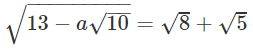Ans.Squaring both side,
13 - a√10 = 8+5+ 2√40
-a√10  = 2√40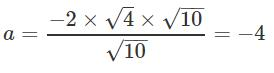a = -4

Q.9. If x + √13 = 4, find the value of x -1/x
Ans.
x + √13 = 4
x= 4 - √13
1/x = 1/ 4 - √13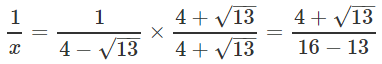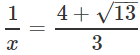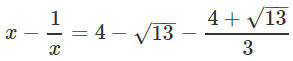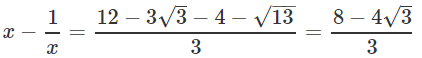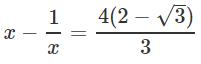Q.10. Find the value of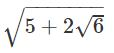Ans.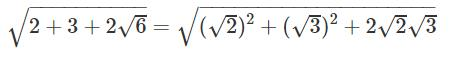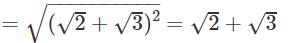Q.11. Simplify (11+√11)(11-√11)
Ans.
112 - 11 = 121 -11 = 110

Q.12. Find x and y if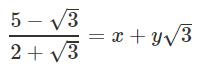Ans.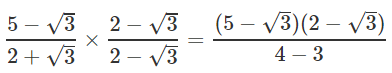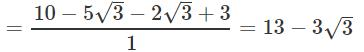x = 13, y = -3

Q.13. find the value of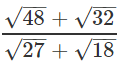Ans.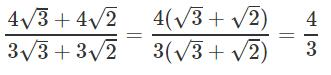Q.14. Simplify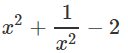if x = √7 + √6
Ans.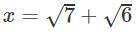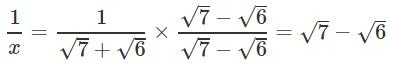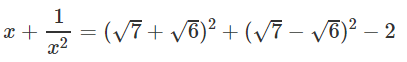= 13 + 2√42 + 13 - 2√42 - 2
= 24

Q.15. If xy = 1 and x = 7 + 4√3, what is the value of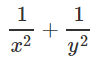Ans.
x = 7 + 4√3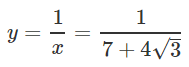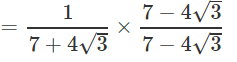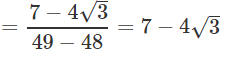1/x = y and y = 1/x,
so,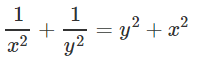= (7 - 4√3)2 + (7 + 4√3)2
= 49-48 - 56√3 + 49 + 48 + 56√3
= 194

Q.16. (2x)2  if 4x - 4x-1 = 24.
Ans.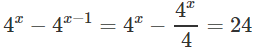suppose 4x = m
m - m/4 = 24
3m/4 = 24
m = (24 x 4)/3
m = 32
∴ 4x = 32
= 22x = 25
= 2x = 5
=x = 5/2

Q. 17. if x = 2 and y = 4, what is value of (x/y)x-y + (y/x)y-x
Ans. (2/4)-2 + (4/2)4-2 = 22 + 22 = 8

Q.18. Simplify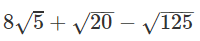Ans.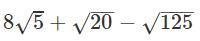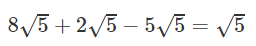Offer running on EduRev: Apply code STAYHOME200 to get INR 200 off on our premium plan EduRev Infinity!

## Mathematics (Maths) Class 9

47 videos|324 docs|82 tests

,

,

,

,

,

,

,

,

,

,

,

,

,

,

,

,

,

,

,

,

,

;